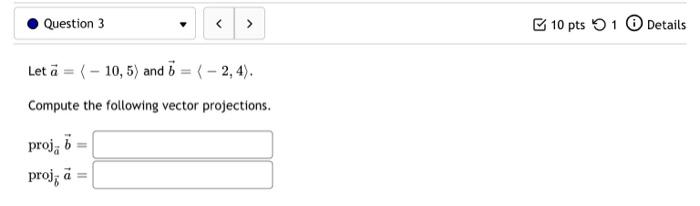### Create an Account

Already have account?

### Forgot Your Password ?

Home / Questions / Question 3 10 pts 1 Details Let a = (-10,5) and 5 = { - 2, 4). Compute the following vecto...

# Question 3 10 pts 1 Details Let a = (-10,5) and 5 = { - 2, 4). Compute the following vector projections. proj; 6 = proj; a =

Question 3 10 pts 1 Details Let a = (-10,5) and 5 = { - 2, 4). Compute the following vector projections. proj; 6 = proj; a =Apr 13 2021 View more View Less

#### Answer (Solved)Subscribe To Get Solution